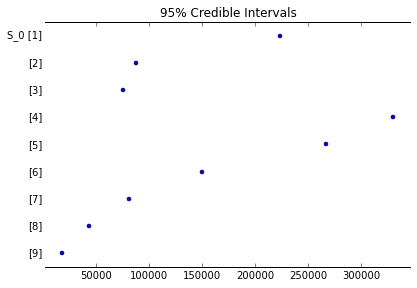# Disease Outbreak Response Decision-making Under Uncertainty: A retrospective analysis of measles in Sao Paulo¶

In :
%matplotlib inline
import pandas as pd
import numpy as np
import numpy.ma as ma
from datetime import datetime
import matplotlib.pyplot as plt
import pdb

from IPython.core.display import HTML
def css_styling():
styles = open("styles/custom.css", "r").read()
return HTML(styles)
css_styling()

Out:
In :
data_dir = "data/"

In :
!rm -rf ~/.theano


Import outbreak data

In :
measles_data = pd.read_csv(data_dir+"measles.csv", index_col=0)
measles_data.BIRTH = pd.to_datetime(measles_data.BIRTH)
measles_data.ONSET = pd.to_datetime(measles_data.ONSET)

In :
measles_data = measles_data.replace({'DISTRICT': {'BRASILANDIA':'BRAZILANDIA'}})


Sao Paulo population by district

In :
sp_pop = pd.read_csv(data_dir+'sp_pop.csv', index_col=0)

In :
_names = sp_pop.index.values
_names[_names=='BRASILANDIA'] = 'BRAZILANDIA'
sp_pop.set_index(_names, inplace = True)

In :
sp_pop.head()

Out:
0 a 4 anos 5 a 9 anos 10 a 14 anos 15 a 19 anos 20 a 24 anos 25 a 29 anos 30 a 34 anos 35 a 39 anos 40 a 44 anos 45 a 49 anos 50 a 54 anos 55 a 59 anos 60 a 64 anos 65 a 69 anos 70 a 74 anos 75 anos e + Total
AGUA RASA 5411 5750 6450 7122 7621 7340 6999 6984 6346 5608 4987 4212 4152 3595 2937 3637 89151
ALTO DE PINHEIROS 2070 2369 2953 3661 4612 4190 3539 3633 3448 3289 3040 2533 2298 1732 1305 1823 46495
ANHANGUERA 3068 3006 2755 2431 2426 2636 2695 2308 1653 1107 753 509 352 217 162 171 26249
ARICANDUVA 7732 7730 8373 8956 9182 8531 7813 7365 6551 5554 4887 3858 3320 2449 1611 1723 95635
ARTUR ALVIM 9031 9078 10000 11058 11387 10347 9125 8658 7830 7055 5919 4612 3756 2633 1727 1724 113940

Annual vaccination data

In :
vaccination_data = pd.read_csv('data/BrazilVaxRecords.csv', index_col=0)

Out:
BIRTHS VAX POP SIA
YEAR
1980 3896442 0.57 121740438 0
1981 3933136 0.73 124610790 0
1982 3952137 0.66 127525420 0
1983 3952735 0.68 130455659 0
1984 3935224 0.73 133364277 0
In :
vaccination_data.VAX[:18]

Out:
YEAR
1980    0.57
1981    0.73
1982    0.66
1983    0.68
1984    0.73
1985    0.67
1986    0.67
1987    0.64
1988    0.62
1989    0.60
1990    0.78
1991    0.85
1992    0.91
1993    0.85
1994    0.77
1995    0.87
1996    0.80
1997    0.99
Name: VAX, dtype: float64
In :
vax_97 = np.r_[*(1979-1921+1), vaccination_data.VAX[:17]]
n = len(vax_97)
FOI_mat = np.resize((1 - vax_97*0.9), (n,n)).T

In :
# Mean age of infection for those born prior to vaccination coverage, assuming R0=16
A = 4.375

In :
(1 - vax_97*0.9)[:-1]

Out:
array([ 1.   ,  1.   ,  1.   ,  1.   ,  1.   ,  1.   ,  1.   ,  1.   ,
1.   ,  1.   ,  1.   ,  1.   ,  1.   ,  1.   ,  1.   ,  1.   ,
1.   ,  1.   ,  1.   ,  1.   ,  1.   ,  1.   ,  1.   ,  1.   ,
1.   ,  1.   ,  1.   ,  1.   ,  1.   ,  1.   ,  1.   ,  1.   ,
1.   ,  1.   ,  1.   ,  1.   ,  1.   ,  1.   ,  1.   ,  1.   ,
1.   ,  1.   ,  1.   ,  1.   ,  1.   ,  1.   ,  1.   ,  1.   ,
1.   ,  1.   ,  1.   ,  1.   ,  1.   ,  1.   ,  1.   ,  1.   ,
1.   ,  1.   ,  1.   ,  0.487,  0.343,  0.406,  0.388,  0.343,
0.397,  0.397,  0.424,  0.442,  0.46 ,  0.298,  0.235,  0.181,
0.235,  0.307,  0.217])
In :
np.tril(FOI_mat).sum(0)

Out:
array([ 64.84 ,  63.84 ,  62.84 ,  61.84 ,  60.84 ,  59.84 ,  58.84 ,
57.84 ,  56.84 ,  55.84 ,  54.84 ,  53.84 ,  52.84 ,  51.84 ,
50.84 ,  49.84 ,  48.84 ,  47.84 ,  46.84 ,  45.84 ,  44.84 ,
43.84 ,  42.84 ,  41.84 ,  40.84 ,  39.84 ,  38.84 ,  37.84 ,
36.84 ,  35.84 ,  34.84 ,  33.84 ,  32.84 ,  31.84 ,  30.84 ,
29.84 ,  28.84 ,  27.84 ,  26.84 ,  25.84 ,  24.84 ,  23.84 ,
22.84 ,  21.84 ,  20.84 ,  19.84 ,  18.84 ,  17.84 ,  16.84 ,
15.84 ,  14.84 ,  13.84 ,  12.84 ,  11.84 ,  10.84 ,   9.84 ,
8.84 ,   7.84 ,   6.84 ,   5.84 ,   5.353,   5.01 ,   4.604,
4.216,   3.873,   3.476,   3.079,   2.655,   2.213,   1.753,
1.455,   1.22 ,   1.039,   0.804,   0.497,   0.28 ])
In :
natural_susc = np.exp((-1/A) * np.tril(FOI_mat).sum(0))[::-1]
vacc_susc = (1 - vax_97*0.9)[::-1]
vacc_susc = 0.5
vacc_susc

Out:
array([ 0.5  ,  0.217,  0.307,  0.235,  0.181,  0.235,  0.298,  0.46 ,
0.442,  0.424,  0.397,  0.397,  0.343,  0.388,  0.406,  0.343,
0.487,  1.   ,  1.   ,  1.   ,  1.   ,  1.   ,  1.   ,  1.   ,
1.   ,  1.   ,  1.   ,  1.   ,  1.   ,  1.   ,  1.   ,  1.   ,
1.   ,  1.   ,  1.   ,  1.   ,  1.   ,  1.   ,  1.   ,  1.   ,
1.   ,  1.   ,  1.   ,  1.   ,  1.   ,  1.   ,  1.   ,  1.   ,
1.   ,  1.   ,  1.   ,  1.   ,  1.   ,  1.   ,  1.   ,  1.   ,
1.   ,  1.   ,  1.   ,  1.   ,  1.   ,  1.   ,  1.   ,  1.   ,
1.   ,  1.   ,  1.   ,  1.   ,  1.   ,  1.   ,  1.   ,  1.   ,
1.   ,  1.   ,  1.   ,  1.   ])
In :
sia_susc = np.ones(len(vax_97))
birth_year = np.arange(1922, 1998)[::-1]
by_mask = (birth_year > 1983) & (birth_year < 1992)

In :
total_susc = sia_susc * vacc_susc * natural_susc
total_susc

Out:
array([  4.69002500e-01,   1.93697440e-01,   2.55462677e-01,
1.85322917e-01,   1.36953286e-01,   1.68513200e-01,
3.99236891e-02,   5.54765276e-02,   4.81834127e-02,
4.19519164e-02,   3.58729698e-02,   3.27610800e-02,
2.61705672e-02,   2.70916231e-02,   1.29180455e-01,
1.00905818e-01,   1.28176341e-01,   2.09416838e-01,
1.66626583e-01,   1.32579684e-01,   1.05489605e-01,
8.39348576e-02,   6.67844030e-02,   5.31383100e-02,
4.22805305e-02,   3.36413269e-02,   2.67673765e-02,
2.12979840e-02,   1.69461555e-02,   1.34835384e-02,
1.07284398e-02,   8.53629188e-03,   6.79206677e-03,
5.40424011e-03,   4.29998882e-03,   3.42136979e-03,
2.72227946e-03,   2.16603463e-03,   1.72344761e-03,
1.37129463e-03,   1.09109726e-03,   8.68152769e-04,
6.90762647e-04,   5.49618743e-04,   4.37314850e-04,
3.47958071e-04,   2.76859611e-04,   2.20288738e-04,
1.75277021e-04,   1.39462573e-04,   1.10966110e-04,
8.82923454e-05,   7.02515229e-05,   5.58969914e-05,
4.44755291e-05,   3.53878203e-05,   2.81570079e-05,
2.24036713e-05,   1.78259171e-05,   1.41835379e-05,
1.12854079e-05,   8.97945446e-06,   7.14467769e-06,
5.68480185e-06,   4.52322323e-06,   3.59899059e-06,
2.86360691e-06,   2.27848457e-06,   1.81292059e-06,
1.44248555e-06,   1.14774170e-06,   9.13223020e-07,
7.26623669e-07,   5.78152263e-07,   4.60018100e-07,
3.66022354e-07])
In :
pd.Series(total_susc).plot();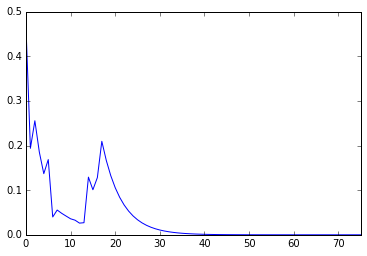Plot of cumulative cases by district

In :
measles_onset_dist = measles_data.groupby(['DISTRICT','ONSET']).size().unstack(level=0).fillna(0)
measles_onset_dist.cumsum().plot(legend=False, grid=False)

Out:
<matplotlib.axes._subplots.AxesSubplot at 0x7f4858c5ba50>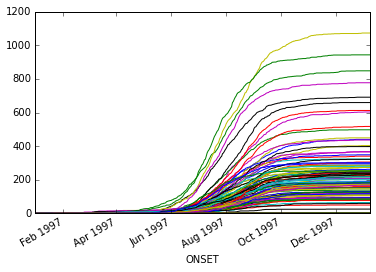In :
total_district_cases = measles_onset_dist.sum()


Top 5 districts by number of cases

In :
totals = measles_onset_dist.sum()
totals.sort(ascending=False)
totals[:5]

Out:
DISTRICT
GRAJAU             1074
JARDIM ANGELA       944
CAPAO REDONDO       849
JARDIM SAO LUIZ     778
CAMPO LIMPO         692
dtype: float64

Age distribution of cases, by confirmation status

In :
by_conclusion = measles_data.groupby(["YEAR_AGE", "CONCLUSION"])
counts_by_cause = by_conclusion.size().unstack().fillna(0)
ax = counts_by_cause.plot(kind='bar', stacked=True, xlim=(0,50), figsize=(15,5))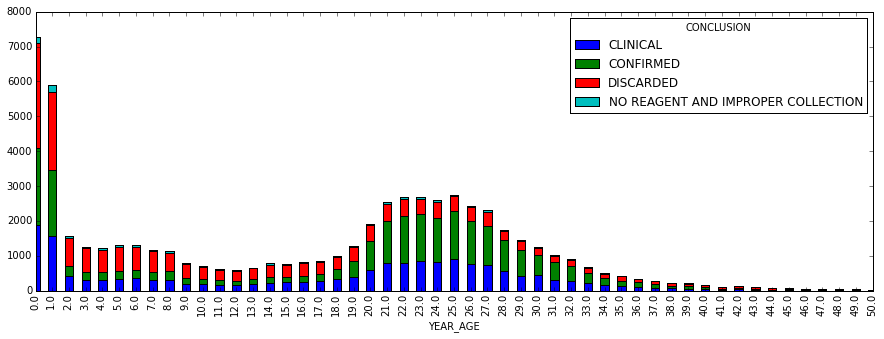## Stochastic Disease Transmission Model¶

As a baseline for comparison, we can fit a model to all the clinically-confirmed cases, regardless of lab confirmation status. For this, we will use a simple SIR disease model, which will be fit using MCMC.

This model fits the series of 2-week infection totals in each district $i$ as a set of Poisson models:

$$Pr(I(t)_{i} | \lambda(t)_i) = \text{Poisson}(\lambda(t)_i)$$

Where the outbreak intensity is modeled as:

$$\lambda(t)_i = \beta [I^{(w)}(t-1)_i]^{\alpha} S(t-1)_i$$

$$\alpha \sim \text{Exp}(1)$$

We will assume here that the transmission rate is constant over time (and across districts):

$$\beta \sim \text{Gamma}(1, 0.1)$$

To account for the influence of infected individuals from neighboring districts on new infections, the outbreak intensity was modeled using a spatial-weighted average of infecteds across districts, where populations were weighted as an exponential function of the distance between district centroids:

$$w_{d} = \text{exp}(-\theta d)$$

$$\theta \sim \text{Exp}(1)$$

### Confirmation Sub-model¶

Rather than assume all clinical cases are true cases, we can adjust the model to account for lab confirmation probability. This is done by including a sub-model that estimates age group-specific probabilities of confirmation, and using these probabilities to estimate the number of lab-confirmed cases. These estimates are then plugged into the model in place of the clinically-confirmed cases.

We specified a structured confirmation model to retrospectively determine the age group-specific probabilities of lab confirmation for measles, conditional on clinical diagnosis. Individual lab confirmation events $c_i$ were modeled as Bernoulli random variables, with the probability of confirmation being allowed to vary by age group:

$$c_i \sim \text{Bernoulli}(p_{a(i)})$$

where $a(i)$ denotes the appropriate age group for the individual indexed by i. There were 16 age groups, the first 15 of which were 5-year age intervals $[0,5), [5, 10), \ldots , [70, 75)$, with the 16th interval including all individuals 75 years and older.

Since the age interval choices were arbitrary, and the confirmation probabilities of adjacent groups likely correlated, we modeled the correlation structure directly, using a multivariate logit-normal model. Specifically, we allowed first-order autocorrelation among the age groups, whereby the variance-covariance matrix retained a tridiagonal structure.

\begin{aligned} \Sigma = \left[{ \begin{array}{c} {\sigma^2} & {\sigma^2 \rho} & 0& \ldots & {0} & {0} \\ {\sigma^2 \rho} & {\sigma^2} & \sigma^2 \rho & \ldots & {0} & {0} \\ {0} & \sigma^2 \rho & {\sigma^2} & \ldots & {0} & {0} \\ \vdots & \vdots & \vdots & & \vdots & \vdots\\ {0} & {0} & 0 & \ldots & {\sigma^2} & \sigma^2 \rho \\ {0} & {0} & 0 & \ldots & \sigma^2 \rho & {\sigma^2} \end{array} }\right] \end{aligned}

From this, the confirmation probabilities were specified as multivariate normal on the inverse-logit scale.

$$\text{logit}(p_a) = \{a\} \sim N(\mu, \Sigma)$$

Priors for the confirmation sub-model were specified by:

\begin{aligned} \mu_i &\sim N(0, 100) \\ \sigma &\sim \text{HalfCauchy}(25) \\ \rho &\sim U(-1, 1) \end{aligned}

Age classes are defined in 5-year intervals.

In :
age_classes = [0,5,10,15,20,25,30,35,40,100]
measles_data.dropna(subset=['YEAR_AGE'], inplace=True)
measles_data['YEAR_AGE'] = measles_data.YEAR_AGE.astype(int)
measles_data['AGE_GROUP'] = pd.cut(measles_data.AGE, age_classes, right=False)


Lab-checked observations are extracted for use in estimating lab confirmation probability.

In :
CONFIRMED = measles_data.CONCLUSION == 'CONFIRMED'
CLINICAL = measles_data.CONCLUSION == 'CLINICAL'


Extract confirmed and clinical subset, with no missing county information.

In :
lab_subset = measles_data[(CONFIRMED | CLINICAL) & measles_data.COUNTY.notnull()].copy()

In :
age = lab_subset.YEAR_AGE.values
ages = lab_subset.YEAR_AGE.unique()
counties = lab_subset.COUNTY.unique()
y = (lab_subset.CONCLUSION=='CONFIRMED').values

In :
_lab_subset = lab_subset.replace({"CONCLUSION": {"CLINICAL": "UNCONFIRMED"}})
by_conclusion = _lab_subset.groupby(["YEAR_AGE", "CONCLUSION"])
counts_by_cause = by_conclusion.size().unstack().fillna(0)
ax = counts_by_cause.plot(kind='bar', stacked=True, xlim=(0,50), figsize=(15,5), grid=False)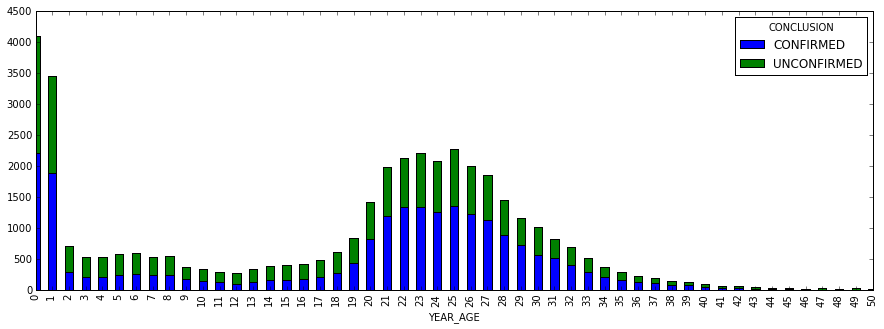Proportion of lab-confirmed cases older than 20 years

In :
(measles_data[CONFIRMED].YEAR_AGE>20).mean()

Out:
0.60257048468117846
In :
#Extract cases by age and time.
age_group = pd.cut(age, age_classes, right=False)
age_index = np.array([age_group.categories.tolist().index(i) for i in age_group])
age_groups = age_group.categories
age_groups

Out:
Index([u'[0, 5)', u'[5, 10)', u'[10, 15)', u'[15, 20)', u'[20, 25)',
u'[25, 30)', u'[30, 35)', u'[35, 40)', u'[40, 100)'],
dtype='object')
In :
# Get index from full crosstabulation to use as index for each district
dates_index = measles_data.groupby(
['ONSET', 'AGE_GROUP']).size().unstack().index

In :
unique_districts = measles_data.DISTRICT.dropna().unique()

In :
excludes = ['BOM RETIRO']

N = sp_pop.drop(excludes).ix[unique_districts].sum().drop('Total')
N

Out:
0 a 4 anos      844130
5 a 9 anos      830880
10 a 14 anos    858750
15 a 19 anos    904972
20 a 24 anos    945244
25 a 29 anos    902086
30 a 34 anos    835888
35 a 39 anos    764605
40 a 44 anos    662946
45 a 49 anos    538872
50 a 54 anos    437744
55 a 59 anos    332195
60 a 64 anos    282850
65 a 69 anos    218202
70 a 74 anos    164842
75 anos e +     203482
dtype: float64
In :
N_age = N.iloc[:8]
N_age.index = age_groups[:-1]
N_age[age_groups[-1]] = N.iloc[8:].sum()
N_age

Out:
[0, 5)        844130
[5, 10)       830880
[10, 15)      858750
[15, 20)      904972
[20, 25)      945244
[25, 30)      902086
[30, 35)      835888
[35, 40)      764605
[40, 100)    2841133
dtype: float64

Calcualte average susceptibility over age groups

In :
age_slice_endpoints = [g[1:-1].split(',') for g in age_groups.values]
age_slices = [slice(int(i), int(i)) for i in age_slice_endpoints]

In :
p_susc_age = np.array([total_susc[s].mean() for s in age_slices])


Compile bi-weekly confirmed and unconfirmed data by Sao Paulo district

In :
sp_counts_2w = lab_subset.groupby(
['ONSET', 'AGE_GROUP']).size().unstack().reindex(dates_index).fillna(0).resample('2W', how='sum')

# All confirmed cases, by district
confirmed_data = lab_subset[lab_subset.CONCLUSION=='CONFIRMED']
confirmed_counts = confirmed_data.groupby(
['ONSET', 'AGE_GROUP']).size().unstack().reindex(dates_index).fillna(0).sum()

all_confirmed_cases = confirmed_counts.reindex_axis(measles_data['AGE_GROUP'].unique()).fillna(0)

In :
# Ensure the age groups are ordered
I_obs = sp_counts_2w.reindex_axis(measles_data['AGE_GROUP'].unique(),
axis=1).fillna(0).values.astype(int)


Check shape of data frame

• 28 bi-monthly intervals, 9 age groups
In :
assert I_obs.shape == (28, len(age_groups))


Prior distribution on susceptible proportion:

$$p_s \sim \text{Beta}(5, 100)$$

In :
from pymc import rbeta
plt.hist(rbeta(5, 100, 10000))

Out:
(array([  6.24000000e+02,   2.75700000e+03,   3.10800000e+03,
2.02200000e+03,   9.60000000e+02,   3.61000000e+02,
1.17000000e+02,   3.90000000e+01,   1.10000000e+01,
1.00000000e+00]),
array([ 0.00393508,  0.02026319,  0.0365913 ,  0.05291941,  0.06924752,
0.08557563,  0.10190374,  0.11823185,  0.13455996,  0.15088807,
0.16721618]),
<a list of 10 Patch objects>)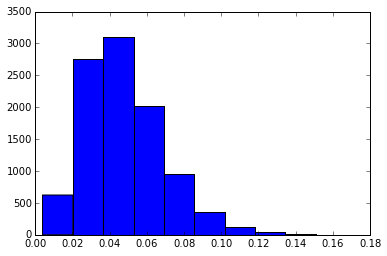In :
I_obs

Out:
array([[   1,    3,    0,    1,    0,    0,    0,    0,    1],
[   4,   13,    7,   18,    1,    2,    0,    1,    4],
[   3,   12,    2,   14,    0,    1,    1,    2,    5],
[   4,   10,    2,   17,    0,    2,    2,    2,    2],
[   6,   15,    7,   19,    1,    3,    1,    7,    6],
[  19,   27,   20,   34,    0,    7,    2,   13,    8],
[   9,   27,    6,   26,    1,    1,    1,    6,    8],
[  13,   27,   13,   20,    1,    4,    2,    5,    2],
[  28,   32,   16,   21,    2,    6,    1,    9,    9],
[  42,   39,   46,   31,    6,   17,    2,   13,   18],
[  93,   69,   72,   40,    4,   18,    6,   19,   26],
[ 157,   95,  153,   64,   12,   47,    5,   31,   42],
[ 359,  183,  315,  169,   26,   95,   18,   76,   68],
[ 807,  363,  622,  282,   65,  234,   34,  162,  136],
[1168,  660, 1035,  388,   87,  398,   63,  257,  166],
[1442,  913, 1193,  536,  137,  430,   48,  318,  292],
[1350, 1051, 1255,  643,  116,  476,   68,  366,  339],
[1314,  933, 1261,  525,  160,  474,   91,  448,  339],
[1218,  773, 1061,  444,  146,  458,   75,  424,  320],
[ 712,  485,  629,  292,   80,  262,   67,  267,  214],
[ 368,  295,  382,  187,   47,  163,   26,  122,   92],
[ 181,  162,  192,  130,   27,   97,   10,   43,   65],
[ 122,  151,   88,  102,   14,   43,   10,   27,   36],
[  72,   95,   63,   64,    6,   36,    2,   15,   18],
[  32,   46,   39,   52,    7,   15,    2,   20,   14],
[  20,   42,   30,   42,    2,    9,    2,    8,   17],
[   7,   23,    5,   15,    1,    4,    3,    3,    7],
[   1,    1,    2,    1,    0,    1,    0,    0,    0]])
In :
# Extract observed data
obs_date = '1997-06-15'
obs_index = sp_counts_2w.index <= obs_date
I_obs_t = I_obs[obs_index]

# Specify confirmation model
confirmation = True

In :
from theano.printing import pp

In [ ]:
from pymc3 import *
import theano.tensor as tt
from theano import shared, scan
from theano.tensor.nlinalg import matrix_inverse as inv
invlogit = tt.nnet.sigmoid

with Model() as model:

n_periods, n_groups = I_obs_t.shape

if confirmation:

mu = Normal('mu', mu=0, tau=0.0001, shape=n_groups)
sig = HalfCauchy('sig', 25, shape=n_groups, testval=np.ones(n_groups))

Tau = tt.diag(tt.pow(sig, -2))

# Age-specific probabilities of confirmation as multivariate normal
# random variables
beta_age = MvNormal('beta_age', mu=mu, tau=Tau,
shape=n_groups)

p_age = Deterministic('p_age', invlogit(beta_age))

p_confirm = invlogit(beta_age[age_index])

# Confirmation likelihood
lab_confirmed = Bernoulli('lab_confirmed', p=p_confirm, observed=y)

# Confirmed infecteds by age
I_conf = Binomial('I_conf', I_obs_t, p_age, shape=I_obs_t.shape)

I = I_conf

else:

I = I_obs_t

# Transmission parameter
beta = HalfCauchy('beta', 25, testval=9)

### Calculate initial number of susceptibles

# Downsample annual series to observed age groups
downsample = lambda x: np.array([x[s].mean() for s in age_slices])

A = Deterministic('A', 75./(beta - 1))
lt_sum = downsample(np.tril(FOI_mat).sum(0)[::-1])
natural_susc = tt.exp((-1/A) * lt_sum)

total_susc = downsample(sia_susc) * downsample(vacc_susc) * natural_susc

# Estimated total initial susceptibles, by age
S_0 = Binomial('S_0', n=shared(N_age.values), p=total_susc, shape=N_age.shape)

# Susceptibles at each time step (subtract cumulative cases from initial S)
S = S_0 - I.cumsum(0)

# Force of infection
lam = tt.transpose(beta * I.sum(1) * tt.transpose(S / N_age.values))

# Likelihood of observed cases as function of FOI of prevous period
like = Potential('like',
dist_math.logpow(lam[:-1], I[1:]) - dist_math.factln(I[1:]) - lam[:-1])

In [ ]:
with model:
step1 = NUTS(vars=model.cont_vars)
step2 = Metropolis(vars=model.disc_vars)
trace = sample(2000, step=(step1, step2))


 [-----            14%                  ] 281 of 2000 complete in 2362.5 sec
In :
burn = 0

In :
traceplot(trace[burn:], vars=['beta', 'A'])

Out:
array([[<matplotlib.axes._subplots.AxesSubplot object at 0x7f47f64417d0>,
<matplotlib.axes._subplots.AxesSubplot object at 0x7f47f63989d0>],
[<matplotlib.axes._subplots.AxesSubplot object at 0x7f47ed845a10>,
<matplotlib.axes._subplots.AxesSubplot object at 0x7f47ed7c6cd0>]], dtype=object)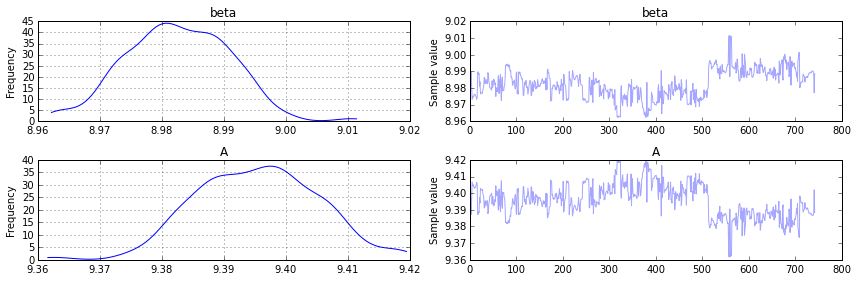In :
forestplot(trace[burn:], vars=['p_age'])

Out:
<matplotlib.gridspec.GridSpec at 0x7f483ddc3bd0>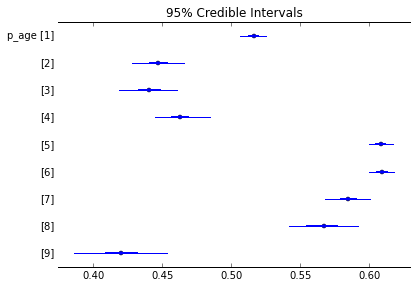In :
forestplot(trace[burn:], vars=['S_0'])

Out:
<matplotlib.gridspec.GridSpec at 0x7f47ed7e8090>